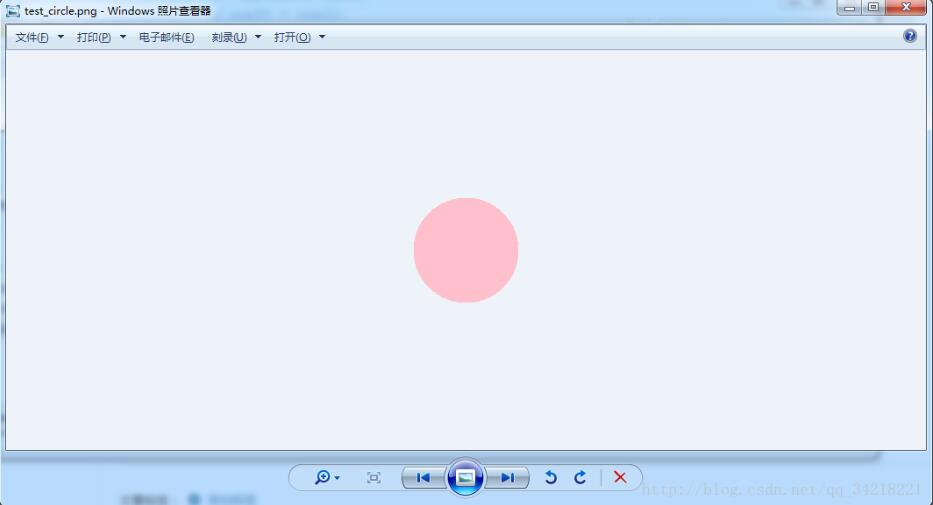### Python编程语言

Python 是一种面向对象、解释型计算机程序设计语言，由Guido van Rossum于1989年底发明，第一个公开发行版发行于1991年。Python语法简洁而清晰，具有丰富和强大的类库。它常被昵称为胶水语言，它能够把用其他语言制作的各种模块（尤其是C/C++）很轻松地联结在一起。

``````
# -*- coding: utf-8 -*-
"""
__author__= 'Du'
__creation_time__= '2018/1/5 9:08'
"""

import os, math
from PIL import Image

def circle():
ima = Image.open("ball1.jpg").convert("RGBA")
# ima = ima.resize((600, 600), Image.ANTIALIAS)
size = ima.size
print(size)

# 因为是要圆形，所以需要正方形的图片
r2 = min(size, size)
if size != size:
ima = ima.resize((r2, r2), Image.ANTIALIAS)

# 最后生成圆的半径
r3 = 60
imb = Image.new('RGBA', (r3*2, r3*2),(255,255,255,0))
r = float(r2/2) #圆心横坐标

for i in range(r2):
for j in range(r2):
lx = abs(i-r) #到圆心距离的横坐标
ly = abs(j-r)#到圆心距离的纵坐标
l = (pow(lx,2) + pow(ly,2))** 0.5 # 三角函数 半径

if l < r3:
pimb[i-(r-r3),j-(r-r3)] = pima[i,j]
imb.save("test_circle.png")

circle() ``````#### 您可能感兴趣的文章:

python生成圆形图片的方法
python生成IP段的方法
Python生成随机数组的方法小结
Python生成随机密码的方法
python处理圆角图片、圆形图片的例子
Python操作生成pdf文件的方法
python实现下载给定网页上图片的方法
Python实现拼接多张图片的方法
Python中暂存上传图片的方法
Python随机生成彩票号码的方法及示例代码
Python实现从订阅源下载图片的方法
python通过pil模块将raw图片转换成png图片的方法